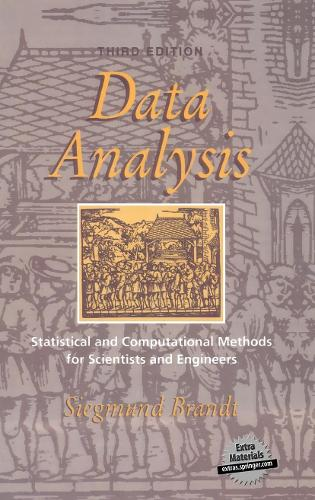•# Data Analysis: Statistical and Computational Methods for Scientists and Engineers (Paperback)

(translator), (author)
£59.99
Paperback 652 Pages / Published: 05/10/2012
• We can order this

Usually dispatched within 15 working days

Bridging the gap between statistical theory and physical experiment, this is a thorough introduction to the statistical methods used in the experimental physical sciences and to the numerical methods used to implement them. The treatment emphasises concise but rigorous mathematics but always retains its focus on applications. Readers are assumed to have a sound basic knowledge of differential and integral calculus and some knowledge of vectors and matrices. After an introduction to probability, random variables, computer generation of random numbers and important distributions, the book turns to statistical samples, the maximum likelihood method, and the testing of statistical hypotheses. The discussion concludes with several important statistical methods: least squares, analysis of variance, polynomial regression, and analysis of time series. Appendices provide the necessary methods of matrix algebra, combinatorics, and many sets of useful algorithms and formulae.

Publisher: Springer-Verlag New York Inc.
ISBN: 9781461271475
Number of pages: 652
Weight: 1044 g
Dimensions: 235 x 155 x 35 mm
Edition: Softcover reprint of the original 3rd ed. 199

MEDIA REVIEWS

From the reviews:

"The book is concise, but gives a sufficiently rigorous mathematical treatment of practical statistical methods for data analysis?It can be of great use to all who are involved with data analysis." Physicalia

"... Serves as a nice reference guide for any scientist interested in the fundamentals of data analysis on the computer." The American Statistician